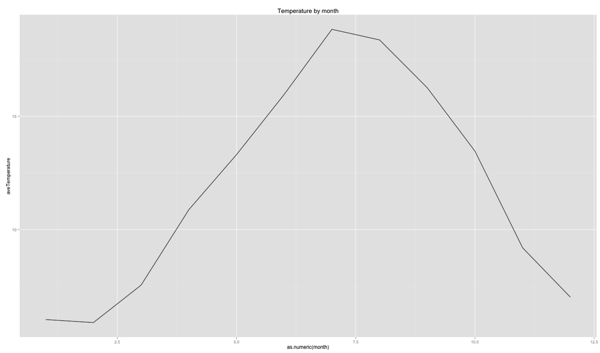# R: ggplot2 - Each group consist of only one observation. Do you need to adjust the group aesthetic?

I’ve been playing around with some weather data over the last couple of days which I aggregated down to the average temperature per month over the last 4 years and stored in a CSV file.

This is what the file looks like:

``````\$ cat /tmp/averageTemperatureByMonth.csv
"month","aveTemperature"
"January",6.02684563758389
"February",5.89380530973451
"March",7.54838709677419
"April",10.875
"May",13.3064516129032
"June",15.9666666666667
"July",18.8387096774194
"August",18.3709677419355
"September",16.2583333333333
"October",13.4596774193548
"November",9.19166666666667
"December",7.01612903225806``````

I wanted to create a simple line chart which would show the months of the year in ascending order with the appropriate temperature.

My first attempt was the following:

``````df = read.csv("/tmp/averageTemperatureByMonth.csv")
df\$month = factor(df\$month, month.name)

ggplot(aes(x = month, y = aveTemperature), data = df) +
geom_line( ) +
ggtitle("Temperature by month")``````

which resulted in the following error:

``geom_path: Each group consist of only one observation. Do you need to adjust the group aesthetic?``

My understanding is that the points don’t get joined up by default because the variable on the x axis is not a continuous one but rather a factor variable.

One way to work around this problem is to make it numeric, like so:

``````ggplot(aes(x = as.numeric(month), y = aveTemperature), data = df) +
geom_line( ) +
ggtitle("Temperature by month")``````

which results in the following chart:This isn’t bad but it’d be much nicer if we could have the month names along the bottom instead.

It turns out we can but we need to specify a group that each point belongs to. ggplot will then connects points which belong to the same group.

In this case we don’t really have one so we’ll define a dummy one instead:

``````ggplot(aes(x = month, y = aveTemperature, group=1), data = df) +
geom_line( ) +
ggtitle("Temperature by month")``````

And now we get the visualisation we want: